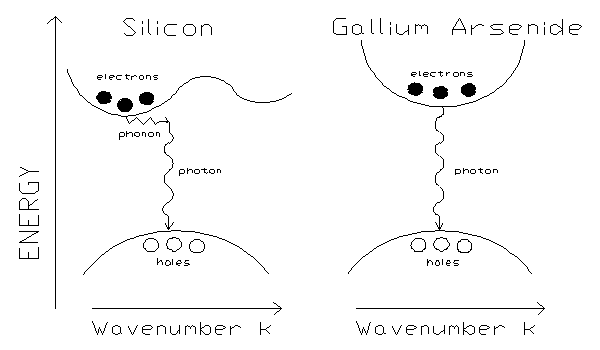# Basics of Semiconductors

Energy Band
In a solid, a group of energy states available to an electron, formed from the interaction of overlapping energy levels from neighboring atoms.
Valence Band
The highest energy band in a solid which is normally completely filled at low temperatures. For semiconductors, it is normally a few electron volts lower in energy than the conduction band.
Conduction Band
A band of energies in which thermally or otherwise excited electrons freely accelerate in the presence of an applied voltage. The conduction band is the lowest energy band which is normally completely empty at low temperatures.
Energy Gap
Prohibited energies between energy bands. In semiconductors it is the difference in energy between the lowest energy state in the conduction band and the highest energy state in the valence band. It forms due to the non-existence of the electron wave functions as solutions to Schrödinger's equation since they interfere destructively rather than constructively at certain energies, as determined by Bragg reflection.Figure 1.1. Direct bandgap of GaAs. (Electronic Archive, Ioffe Physico-Technical Institute)
Momentum Space
An alternate coordinate system used to describe a particle. It is different from the more common position space in that each axis represents a direction for a momentum vector (e.g. the coordinate (4,3) in momentum space would indicate the particle has slightly more momentum in the x-direction than in the y-direction).Figure 1.2. Direct vs. Indirect bandgaps. (Physics Department, University of Oxford)
Direct vs. Indirect Bandgaps
In momentum space, when the lowest-energy point of the conduction band lies directly above the highest-energy point of the valence band in a semiconductor, the movement of a hole or electron across the bandgap conserves momentum and the gap is classified as "direct" (see Eg in Figure 1.1 and Gallium Arsenide in Figure 1.2). For indirect bandgaps the highest-energy point of the valence band is not directly below the lowest-energy point in the conduction band (see Silicon in Figure 1.2) so a phonon must carry away the momentum off-set if a transition is to occur between the valence and conduction band. This causes optical transitions to be much less likely in materials with indirect versus direct bandgaps.
III-V Semiconductors
A group of semiconductors formed by combining an element from column III and column V of the periodic table. Depending on the doping, the semiconductor's main charge carriers may either be electrons (n-doped) or holes (p-doped) and results in the Fermi energy being shifted toward the conduction band (n-doped) or the valence band (p-doped). The optical study of III-V semiconductors is feasible since most have direct energy gaps. This makes the study of electron spin through optical means possible since the band structure has a structure conducive to optical excitation, which can span an energy gap but carries minimal momentum.
Gallium Arsenide (GaAs)
III-V semiconductor with a direct bandgap of approximately 1 eV. GaAs has applications in lasers as the semiconductor element in laser diodes used to optically pump other lasers. The first red and infrared light emitting diodes (LEDs) were developed with GaAs. Since LEDs are designed to emit light instead of having energy carried away by crystal lattice vibrations (phonons), semiconductors with direct (versus indirect) bandgaps must be used.
Effective Mass (m*)
The mass (of an electron or hole in solid states physics) calculated from the curvature of the conduction/valence bands. In a plot of energy versus wavenumber (E vs. k), a band of high curvature corresponds to a small effective mass whereas a band with smaller curvature corresponds to a large effective mass. The effective mass can be found from calculating $\frac{d^2 E}{d k^2}$, and since $\frac{d^2 E}{d k^2}$ can be negative and is proportional to m*, the effective mass may take on negative values (this is often labeled as a hole).
Hole
A missing electron in the valence band of a solid. Holes move in the opposite direction of electrons since they are formed as an electron moves to fill a hole. Although holes are assigned a charge of +e, they are not positrons (electron antimatter). The annihilation of an electron-positron pair produces gamma rays whereas the recombination of an electron-hole pair produces a photon with the energy of the bandgap (EG). Where electrons are the charge carriers in the conduction band, holes are the charge carriers in the valence band and often have larger effective masses than electrons in semiconductors.
An intrinsic property of elementary particles, often thought of as the particle rotating around its axis (which is allowed mathematically.) Spin may take on half-integer values (±½, etc.) and for charged particles it differs from the magnetic dipole moment by a factor of $\frac{-g \times spin}{\hbar}$.